[TOC]

# 1. 1d Range Search

Goal: intersections of geometric objects.Solution: BST

operations required:

• insert
• search
• delete
• range search: all keys between k1 and k2
• range count: how many keys are between k1 and k2

→ find points on an interval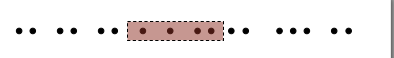## implementation by BST

range count
using the `rank()` function for the BST (or use the size of a tree)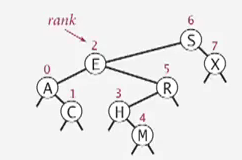```public int size(Key hi, Key lo){
if(contains(hi)) return rank(hi)-rank(lo)+1;
else return rank(hi) - rank(lo);
}
```

range search

• find in left subtree (if could fall into range)
• check current node
• find in right subtree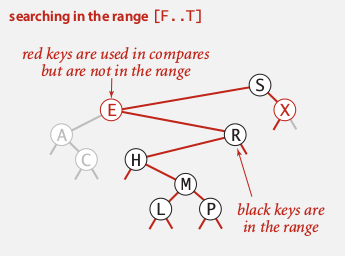running time: R+lgN (R=nb of nodes in range)

# 2. Line Segment Intersection

Orthognal line segment intersection search:
find all intersections given N horizontal/vertical linesNon-degeneracy assumption: all x-coord and y-coord are distinct.

naive algo: check all pairs...

## Sweep-line algorithm• sweep a vertical line from left to right.
• when hit the left end of horizontal-segment (h-seg) → insert into a BST
• when hit the right end of a h-seg → delete from BST
• when hit a vertical-seg: ⇒ 1d range search !

proposition
running time is NlgN+R (R=nb of intersections).
proof.

• Sort by x-coord (or use PQ) → NlgN
• insert/delete y-coord to BST → NlgN
• range search → NlgN + R

# 3. Kd-trees

An extension of BST: 2d-keys.

• insert: insert 2d points
• search
• range search: find all keys lying in a 2d rectangle (h-v rectangle)
• range count

## gird implementation

divide space into a M-by-M grid (uniform squares).
space: N + M^2
time: 1 + N/M^2
→ choose square to balance space and time.
problem: points are not uniformly distributed.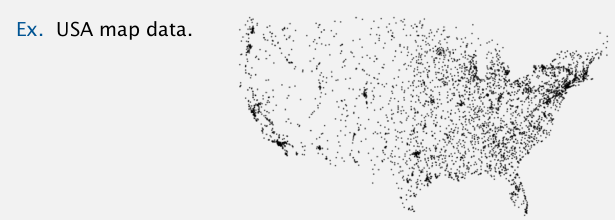## 2d tree

Use a tree to represent the subdivision of the space.

2d tree: recursively divide the space into 2 halfplanes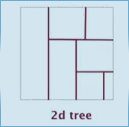construct the 2d tree by adding points: alternating between horizontal and vertical partitioning for each level of tree.Data structure: BST alternating x and y-coords as key.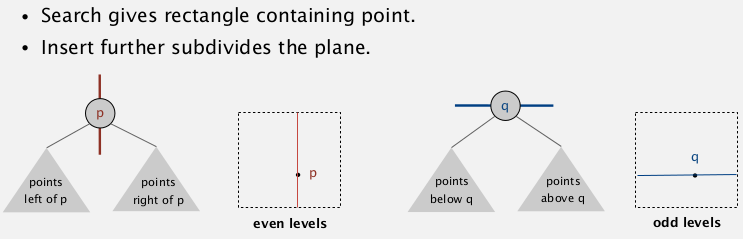## Range search for 2d tree

find all points lying in a rectangle.

• check point in node
• find in left subtree (if could be in range — the rectangle intersects the splitting line)
• find in right subtree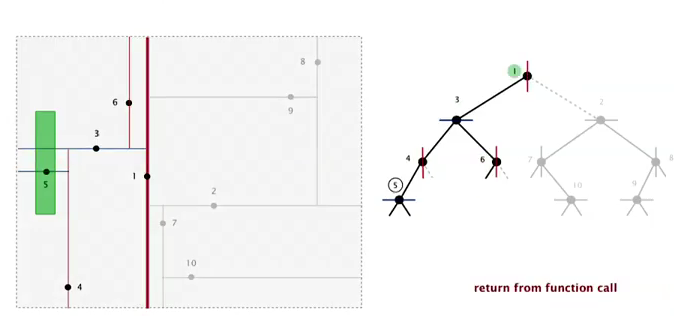analysis
Typical case: R + lgN
worst case: R+ sqrt(N) (even if tree is balanced)
(proof is hard)

## Nearest Neighbour seach

find closest point to a query point.

• check dist from query point to node
• check in left tree (if could contain a closer point — 和两点连线与splitting line的角度有关系)
• check in right tree

analysis
typical case: lgN
worst case: N

Flocking boids
3 simple rules to get a simulation of flocking.## Kd tree

partition the k-dim space into 2 halfspaces.
cycle through k dimensions.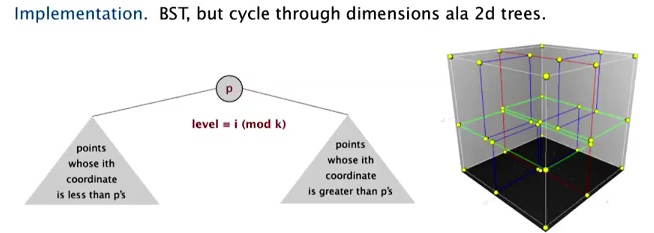(居然时一个本科生发现的!)

Nbody simulation:
treat clusters as an aggregated node

# 4. Interval search tree

1d interval search:　data are intervals

• insert interval
• search interval
• delete interval
• intersection query: find all intervals that intersects (lo,hi)Nondegeneracy assumption: all left endpoint of intervals are distinct.

API:

```put(Key lo, Key hi, Value val)
get(Key lo, Key hi)
delete(Key lo, Key hi)
Iterable<Key> intersects(Key lo, Key hi)
```

## Interval search tree:

• BST using left endpoint as key
• in each node: store the max right endpoint of the subtree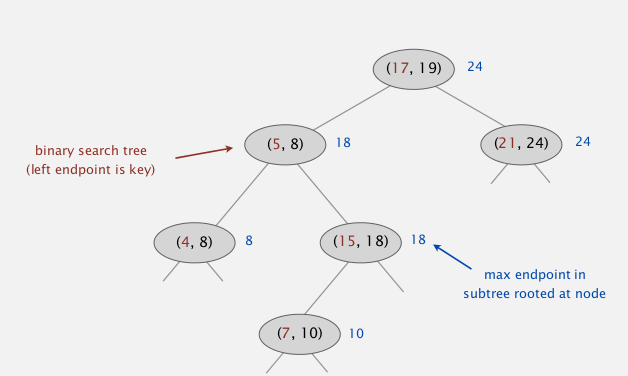insert

search
search any one interval that intersects (lo,hi)

• if node intersects, return
• if left.maxendpoint < lo: go right
• else: go left

*proof. *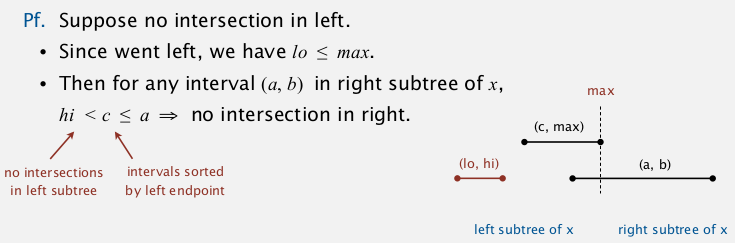# 5. Rectangle intersection

Goal: find all intersection among N rectangles.
(non degeneracy assumption: all x and y are distinct)

bottom line: linearithmic algo.

sweep-line algorithm:
sweep vertical line from left to right.

• when hit left part of a rect ⇒ put into an interval search tree
• when hit right part of a rect ⇒ remove interval
• every time befor adding ⇒ check intersection

reduces the 2d rect intersection pb to 1d interval search pb.

complexity:
NlgN+RlgN

summery: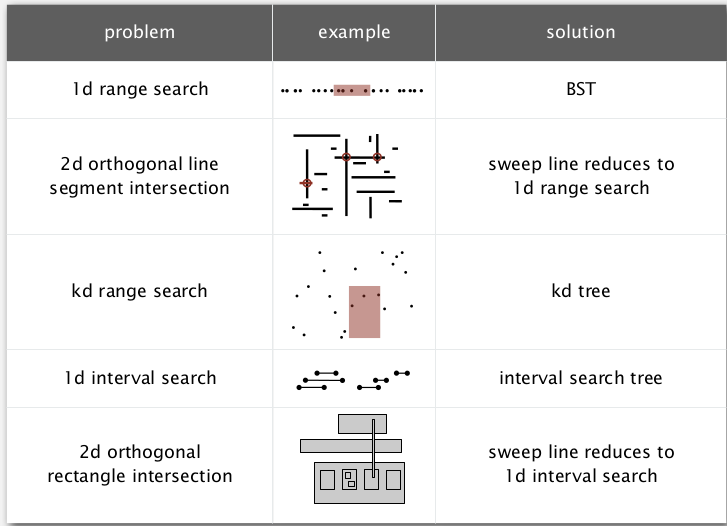#### Part 12 of series «Algorithms Princeton MOOC I»：

comments powered by Disqus